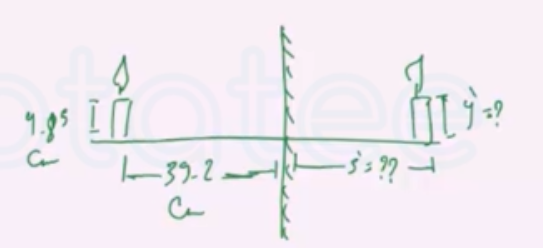Need Help?

Subscribe to Physics 2

###### \${selected_topic_name}
• Notes

$\begin{array}{l}{\text { A candle } 4.85 \mathrm{cm} \text { tall is } 39.2 \mathrm{cm} \text { to the left of a plane mir- }} \\ {\text { ror. Where is the image formed by the mirror, and what is the }} \\ {\text { height of this image? }}\end{array}$

$y=4.85 \mathrm{cm}$

$S=39.2 \mathrm{cm}$

$S^{\prime}=? ? \quad , y^{\prime}=??$$S=-S' \Rightarrow S^{\prime}=-S$$= - 39.2 \mathrm{cm}$

$M=\frac{y^{\prime}}{y}=1$$\Rightarrow 1=\frac{y^{\prime}}{4.85} \Rightarrow y'=4.85 cm$